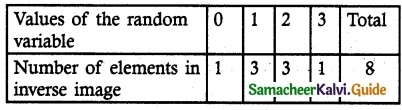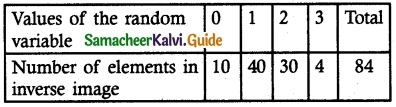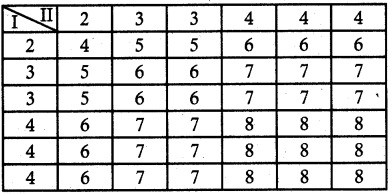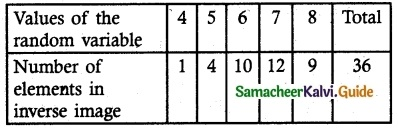# Samacheer Kalvi 12th Maths Guide Chapter 11 Probability Distributions Ex 11.1

Tamilnadu State Board New Syllabus Samacheer Kalvi 12th Maths Guide Pdf Chapter 11 Probability Distributions Ex 11.1 Textbook Questions and Answers, Notes.

## Tamilnadu Samacheer Kalvi 12th Maths Solutions Chapter 11 Probability Distributions Ex 11.1

Question 1.
Suppose X is the number of tails occurred when three fair coins are tossed once simultaneously. Find the values of the random variable X and number of points in its. inverse – images.
Solution:
Let X be the random variable of number of tails when three coins tossed.
∴ X = {0, 1, 2, 3}
S = {HHH, HHT, HTH, THH, HTT, THT, TTH, TTT}
n (S) = 8
X-1 ({0}) = {TTT}
X-1 ({1}) = {HHT, HTH, THH}
X-1 ({2}) = {HTT, THT, TTH}
X-1 ({3}) = {HHH}Question 2.
In a pack of 52 playing cards, two cards are drawn at random simultaneously. If the number of black cards drawn is a random variable, find the values of the random variable and number of points in its inverse images.
Solution:
Let X be the random variable of number of black cards occur.
∴ X = {0, 1, 2}
The sample space consists of 52C2 = 1326
X = 0, X (both are white cards) = 26C2 = 325
X = 1, X (one black and one white cards)
= 26C1 × 26C1
= 676
X = 2, X (both are black cards) = 26C2 = 325Question 3.
An urn contains 5 mangoes and 4 apples. Three fruits are taken at random. If the number of apples taken is a random variable, then find the values of the random variable and number of points in its inverse images.
Solution:
Let X be the random variable of getting apples
Given 5 mangoes and 4 apples are in an urn
∴ X = {0, 1, 2, 3}
The sample space consists of 9C3 = 84
X = 0, X (3 mangoes) = 5C3 = 10
X = 1, X (2 mangoes and 1 apple)
= 5C3 × 4C1 = 40
X = 2, X (1 mango and 2 apples)
= 5C1 × 4C2 = 30
X = 3, X (3 apples) = 4C3 = 4Question 4.
Two balls are chosen randomly from an urn containing 6 red and 8 black balls. Suppose that we win Rs 15 for each red ball selected and we lose Rs 10 for each black ball selected. If X denotes the winning amount, then find the values of X and number of points in its inverse images.
Solution:
Let X be the random variable denotes the j winning amount.
X (Both are black balls) = Rs 2 (-10) = -Rs 20
X (one red and one black ball) = Rs 15 – Rs 10 = Rs 5
X (both are red ball) = Rs 2 (15) = Rs 30
∴ X = {-20, 5, 30}
The sample space consists of 14C2 = 91
X = – 20, Both black balls = 8C2 = 28
X = 5, One black, one red ball = 8C1 × 6C1
= 8 × 6 = 48
X = 30, Both are white balls = 6C2 = 15Question 5.
A six sided die is marked ‘2’ on one face, ‘3’ on two of its faces and ‘4’ on remaining three faces. The die is thrown twice. If X denotes the total score in two throws, find the values of the random variable and number of points in its inverse images.
Solution:
Let X be the random variable denotes the total score is two throws of a die.
Sample Space Sn (S) = 36
X = {4, 5, 6, 7, 8}
From the sample spaceScroll to Top